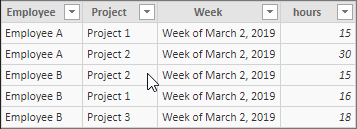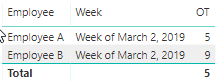cancel
Showing results for
Did you mean:Regular Visitor

## Calculating over time hours for employees

Hi

I need some help calculatin over time hours in a week if the total hours for an employee go over 40 hours in a week. The issue is that employees log hours by project, and can work on more than one project in the same week. My Power BI table may have two rows for one employee for a week if he worked on two projects. So here's an example:

Employee A, Project 1, Week of March 2, 2019, 15 hours

Employee A, Project 2, Week of March 2, 2019, 30 hours

The Over time hours correctly calculated should be OT = (30 + 15) - 40 = 5 hours for this Employee 1.

How can I achieve this?

1 ACCEPTED SOLUTIONSolution Sage

Hi @ms007 ,

If you want to add up total OT hours for all employees in every week, we can use the following one:

```OT =
CALCULATE (
SUM ( Table1[hours] ),
FILTER (
ALL ( Table1 ),
Table1[Week] = MIN ( Table1[Week] )
)
) - 40```

Then only drag the measure and week to the visual.

Best Regards,

Teige

4 REPLIES 4Solution Sage

Hi @ms007 ,

Assume that we have a table like below:We can create a measure like below:

```OT =
CALCULATE (
SUM ( Table1[hours] ),
FILTER (
ALL ( Table1 ),
Table1[Employee] = MIN ( Table1[Employee] )
&& Table1[Week] = MIN ( Table1[Week] )
)
) - 40```

The result will like below:Best Regards,

TeigeResolver I

Hi, this calculation worked perfectly for what I needed, but did not resolve the whole problem. In my case employees can have different contracted hours. So instead of - 40 I would want to substract the individual contracted hrs (like 40 hrs in some cases and 34 ,25 or15 hrs etc in other cases ). That is I need to be able to substract a column (say 'contracted_hrs'). But how can I do this if 'contracted_hrs' is not summed, and I have a Calculate in the first part?Regular Visitor

Hi Teige

Thnk you for your reply. This works. But I have one question. I want to add up the total OT hours for all employees. So how do I do that?Solution Sage

Hi @ms007 ,

If you want to add up total OT hours for all employees in every week, we can use the following one:

```OT =
CALCULATE (
SUM ( Table1[hours] ),
FILTER (
ALL ( Table1 ),
Table1[Week] = MIN ( Table1[Week] )
)
) - 40```

Then only drag the measure and week to the visual.

Best Regards,

Teige